Question
How to prove G（n）=n+1 in this algorithm？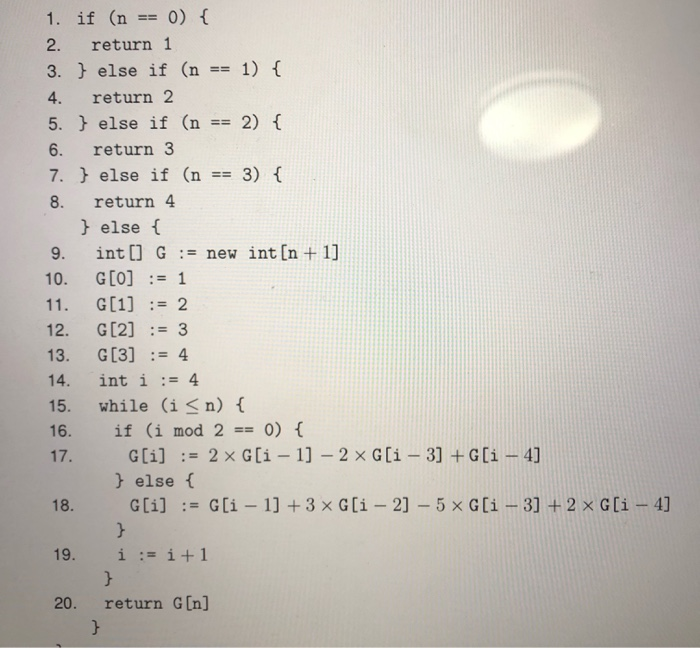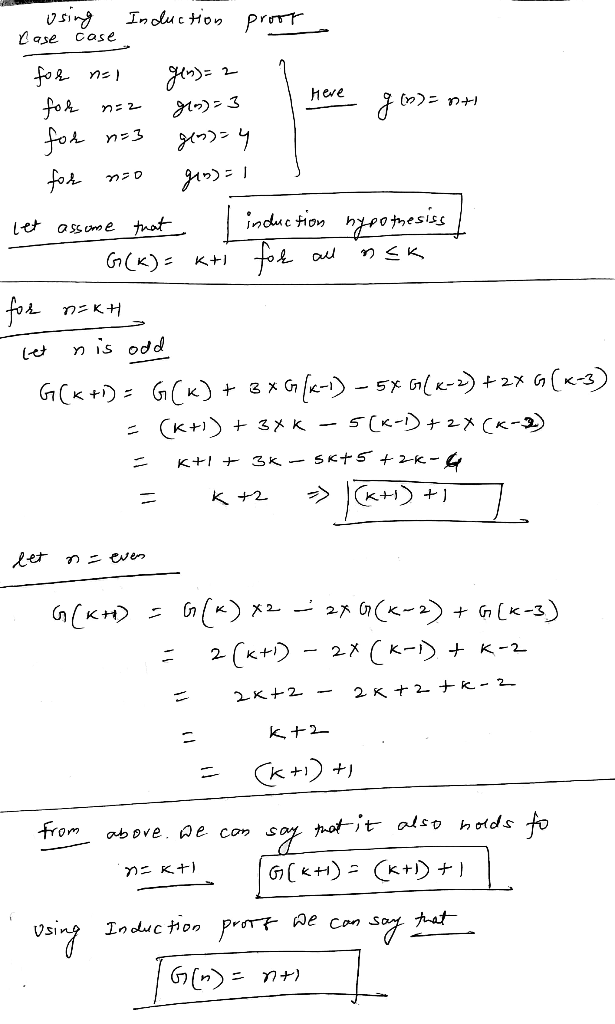#### Earn Coins

Coins can be redeemed for fabulous gifts.

Similar Homework Help Questions
• ### Question 18 CLO3 Analyze the following code and answer the questions that follow def F(n): If n <= 1: return n else: return F(n-1)+F(n-2) for i in range (n) print (F(i)) Result: 0 1 1 2 3 5 8...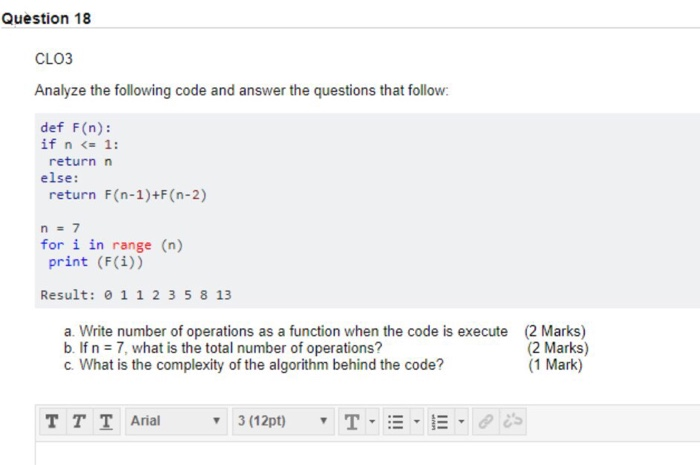Question 18 CLO3 Analyze the following code and answer the questions that follow def F(n): If n <= 1: return n else: return F(n-1)+F(n-2) for i in range (n) print (F(i)) Result: 0 1 1 2 3 5 8 13 a. Write number of operations as a function when the code is execute b If n 7, what is the total number of operations? c. What is the complexity of the algorithm behind the code? (2 Marks) (2 Marks) (1...

• ### 3. Recursive Program (6 points) Consider the following recursive function for n 1: Algorithm 1 int...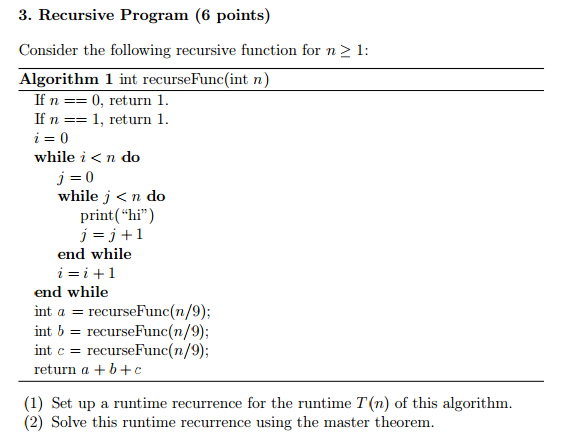3. Recursive Program (6 points) Consider the following recursive function for n 1: Algorithm 1 int recurseFunc(int n) If n 0, return 1. If n 1, return 1 while i< n do while j <n do print("hi") j 1 end while i i 1 end while int a recurse Func(n/9); int b recurse Func (n/9) int c recurse Func (n/9) return a b c (1) Set up a runtime recurrence for the runtime T n) of this algorithm. (2) Solve...

• ### b) Consider the following code. public static int f(int n) if (n == 1) return 0;...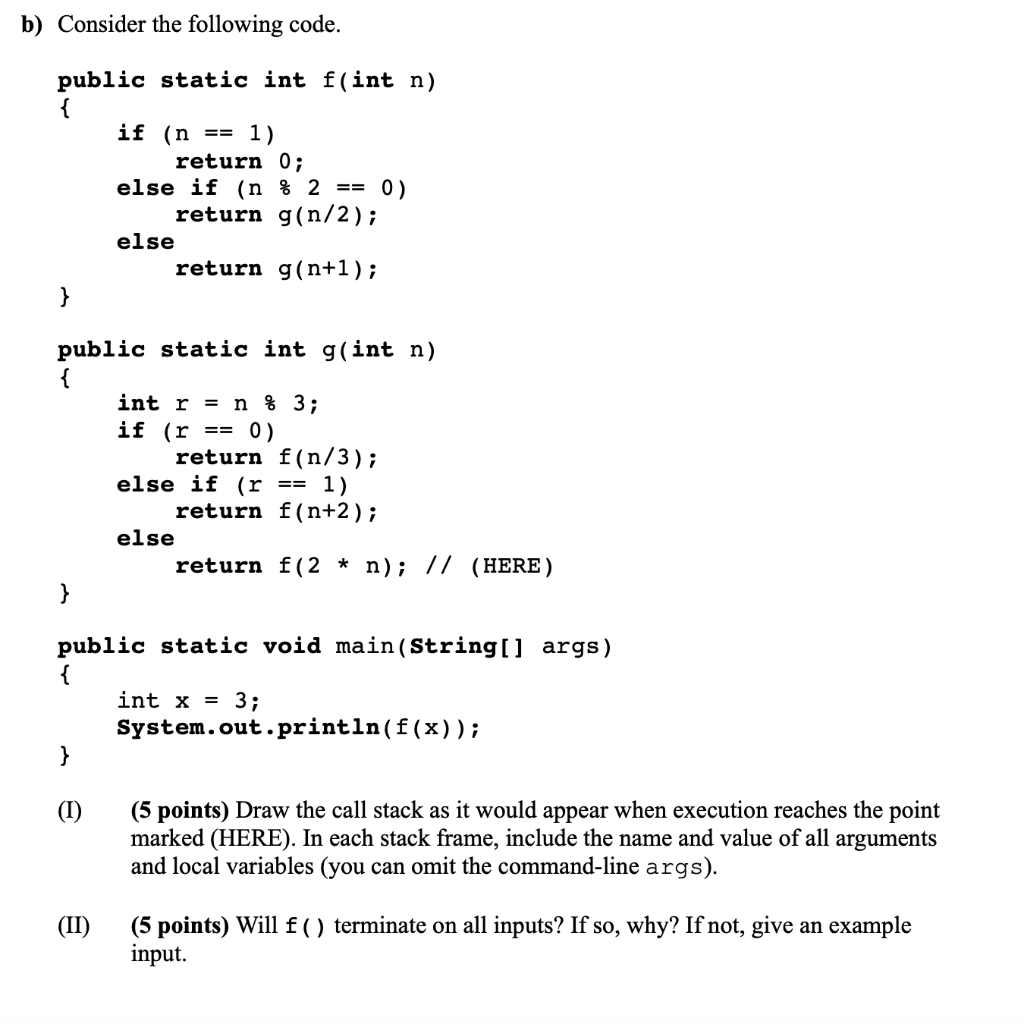b) Consider the following code. public static int f(int n) if (n == 1) return 0; else if (n % 2 == 0). return g(n/2); else return g(n+1); public static int g(int n) int r = n % 3; if (r == 0) return f(n/3); else if (r == 1) return f(n+2); else return f(2 * n); // (HERE) public static void main(String[] args) { int x = 3; System.out.println(f(x)); (1) (5 points) Draw the call stack as it would...

• ### f(t) -S -8 -7 -3 --1 3 5 1 2 13 4 7 8 9 12...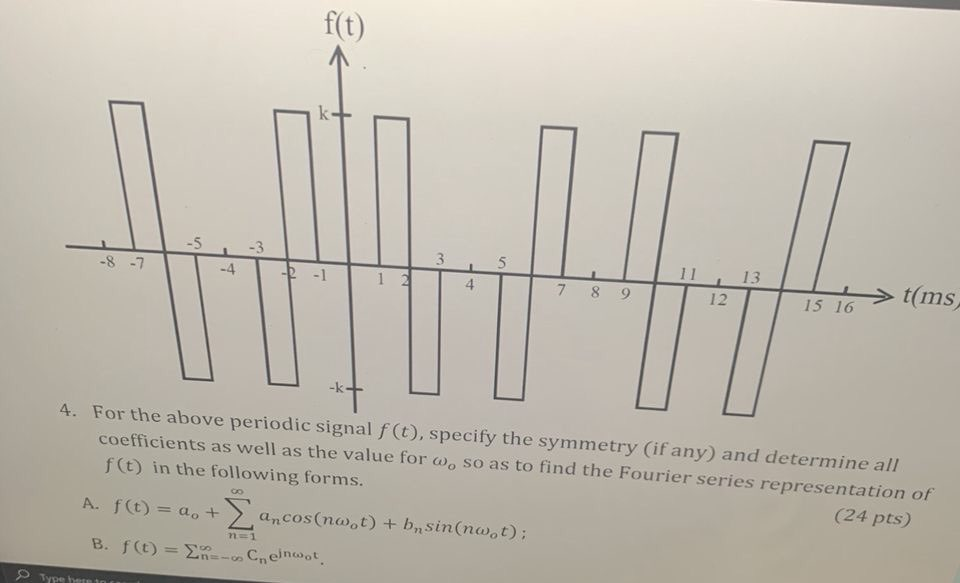f(t) -S -8 -7 -3 --1 3 5 1 2 13 4 7 8 9 12 t(ms 15 16 4. For the above periodic signal f(t), specify the symmetry (if any) and determine all coefficients as well as the value for w, so as to find the Fourier series representation of f(t) in the following forms. (24 pts) GO A. f(t) = a + ancos(not) + b sin(nw.t): B. f(t) = R-Cneinwor. n=1 Type A

• ### 3) [16 points totall Consider the following algorithm: int SillyCalc (int n) { int i; int Num, answer; if (n &lt...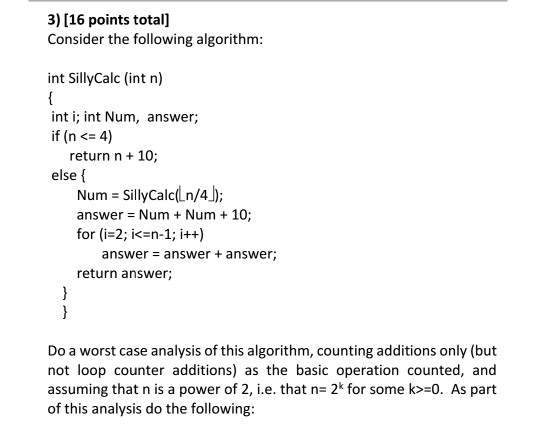3) [16 points totall Consider the following algorithm: int SillyCalc (int n) { int i; int Num, answer; if (n < 4) 10; return n+ else f SillyCalc(Ln/4) answer Num Num 10 for (i-2; i<=n-1; i++) Num + = answer + answer; answer return answer } Do a worst case analysis of this algorithm, counting additions only (but not loop counter additions) as the basic operation counted, and assuming that n is a power of 2, i.e. that n- 2*...

• ### For questions 10-12, refer to the following iterative code computes values for the table t. 1 pub...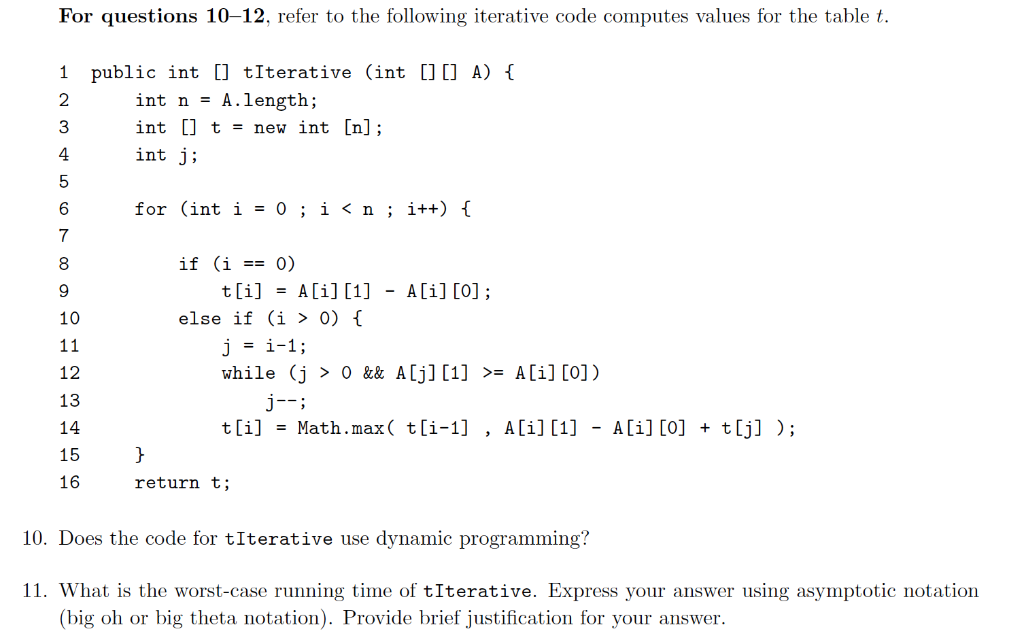For questions 10-12, refer to the following iterative code computes values for the table t. 1 public int [] tIterative (int [C A)1 2 3 4 int n - A.length; int [] t = new int [n]; int j; for (inti-0; i 0) while (j > 0 && A[j]  A[i] [O]) 12 13 14 15 16 t[i]Math.max( t[i-1] , A[i][i] - A[i] [o] + t[j] ); return t; 10. Does the code for tIterative use dynamic programming? 11. What...

• ### 9. (5 points) Please describe an algorithm that takes as input a list of n integers...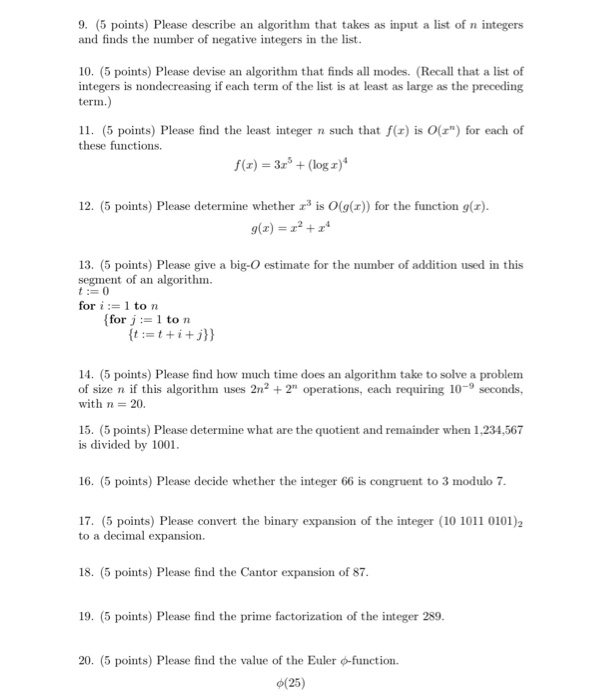9. (5 points) Please describe an algorithm that takes as input a list of n integers and finds the number of negative integers in the list. 10. (5 points) Please devise an algorithm that finds all modes. (Recall that a list of integers is nondecreasing if each term of the list is at least as large as the preceding term.) 11. (5 points) Please find the least integer n such that f() is 0(3") for each of these functions f()...

• ### Compute the sign of the following permutations: (a) (1, 4, 3, 6, 7)(5, 8, 9, 10). (b) σ ∈ Sn, i 7...

Compute the sign of the following permutations: (a) (1, 4, 3, 6, 7)(5, 8, 9, 10). (b) σ ∈ Sn, i 7→ n + 1 − i. (c) Show that this initial configuration of the 15 puzzle is not solvable 3 1 15 13 2 8 5 9 12 7 11 4 14 6 10 − (d) Suppose that (i, j) ∈ Sn is a transposition and that i < j. Find an expression (in terms of i and j...

• ### 1) How many times is the fib method invoked for fb(5)7 a. 14 b. 15 c....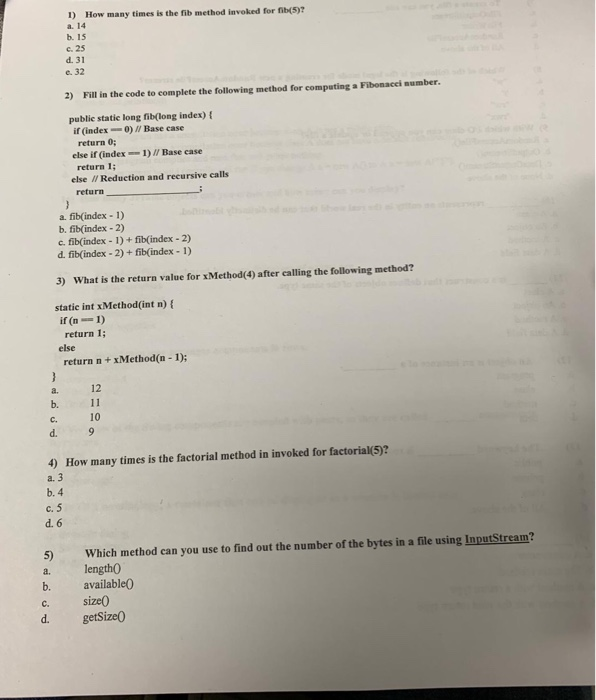1) How many times is the fib method invoked for fb(5)7 a. 14 b. 15 c. 25 d. 31 e. 32 2) Fill in the code to complete the following method for computing Fibonacei aumber publie statie long fib(long index) t if (index--0) Base case return 0; return 1 return else if (index1)/ Base case else I/ Reduction and recursive calls a. fib(index 1) b. fib(index-2) c. fib(index-1)+ fib(index -2) d. fib(index - 2) + fib(index -1) 3) What is...

• ### 1 #include <stdio.h> 2 3 11 Remember this written by the 4 // "new guy down...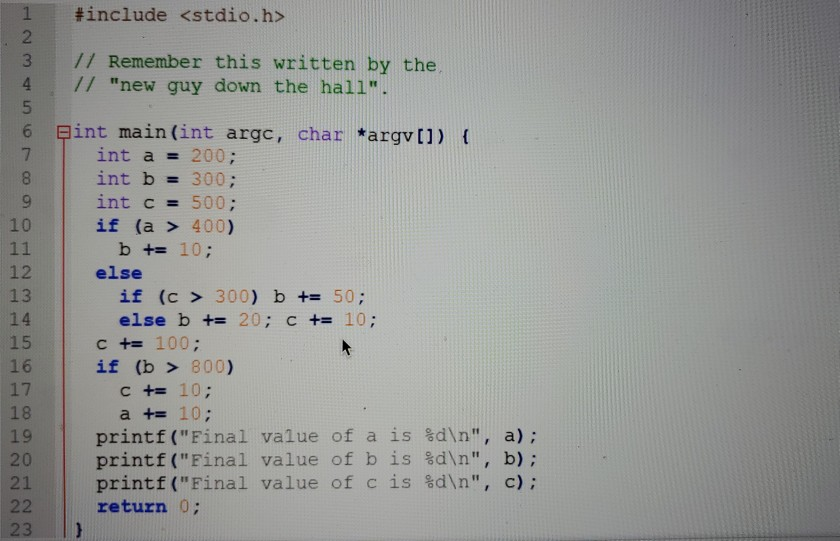1 #include <stdio.h> 2 3 11 Remember this written by the 4 // "new guy down the hall". 5 6 pint main(int argc, char *argv[]) { 7 int a = 200; 8 int b = 300; 9 int c = 500; 10 if (a > 400) 11 b += 10; 12 else 13 if (c > 300) b += 50; 14 else b += 20; C += 10; 15 C += 100; 16 if (b > 800) 17 C +=...